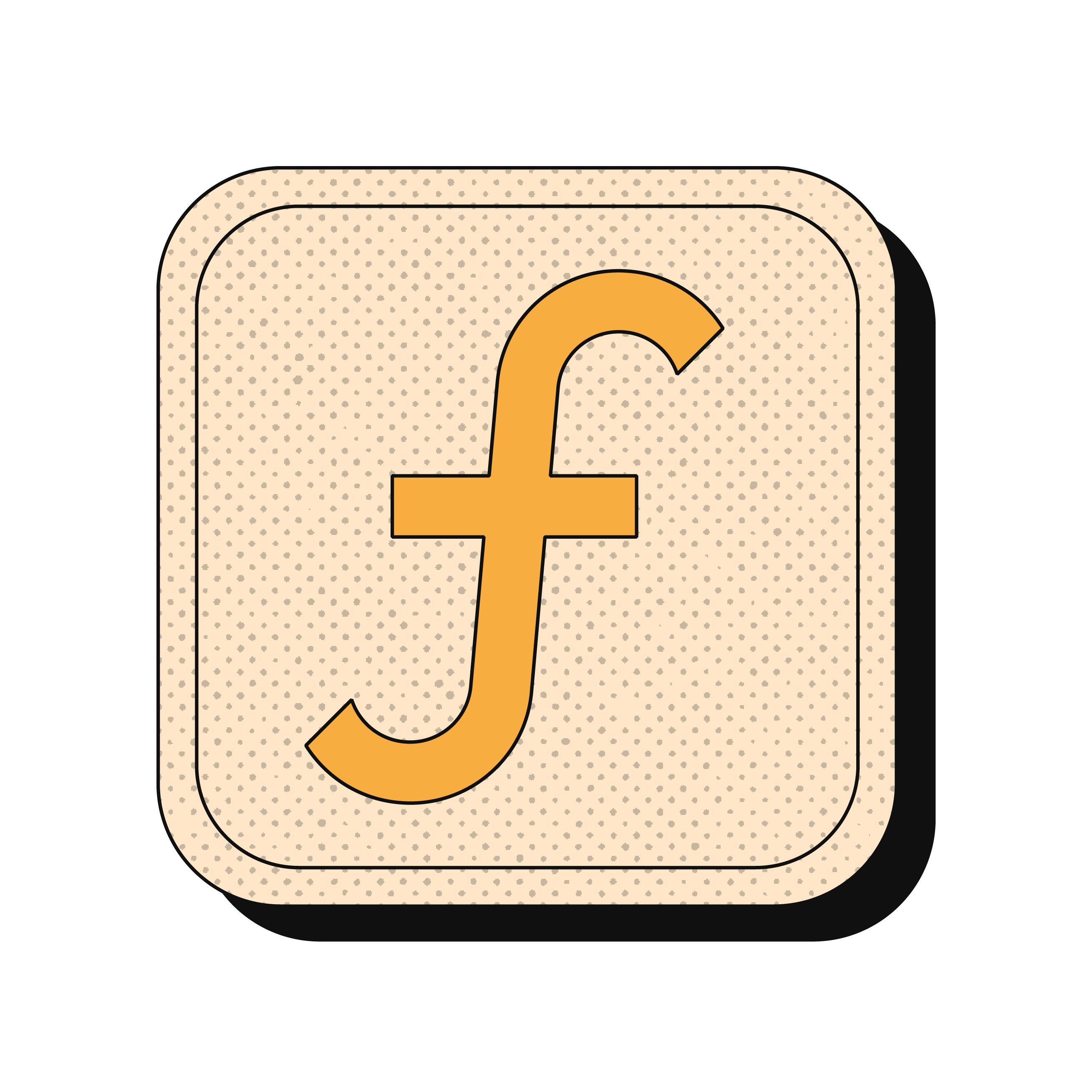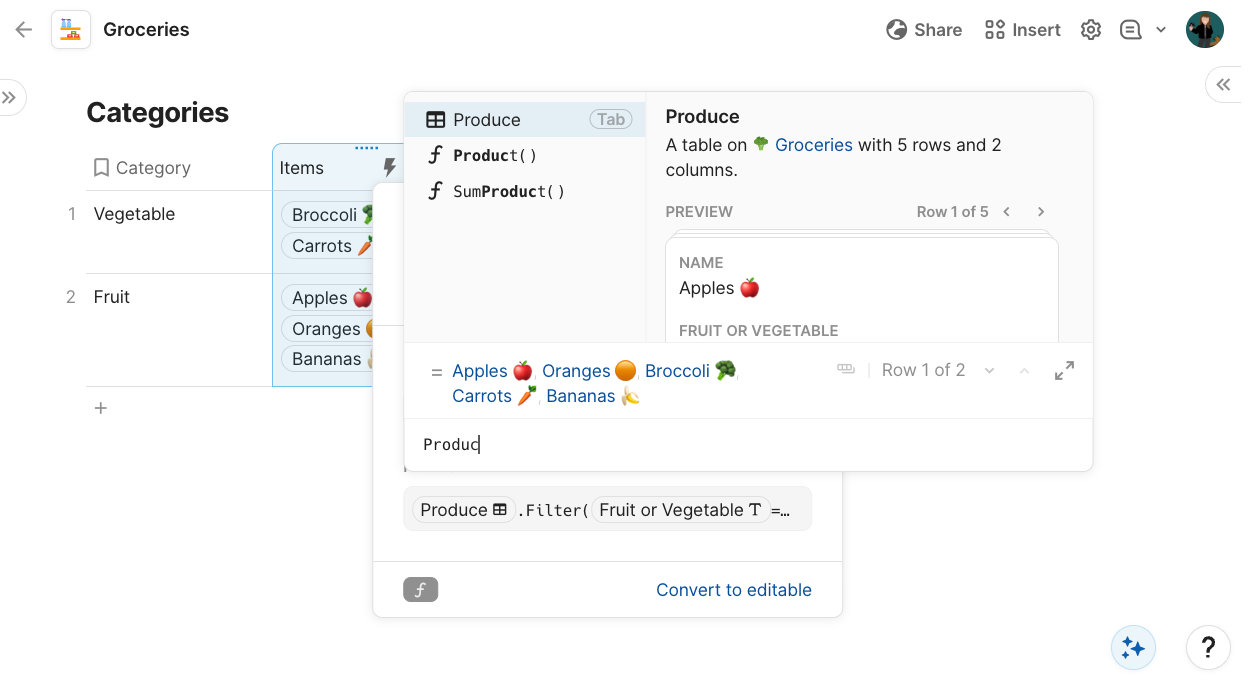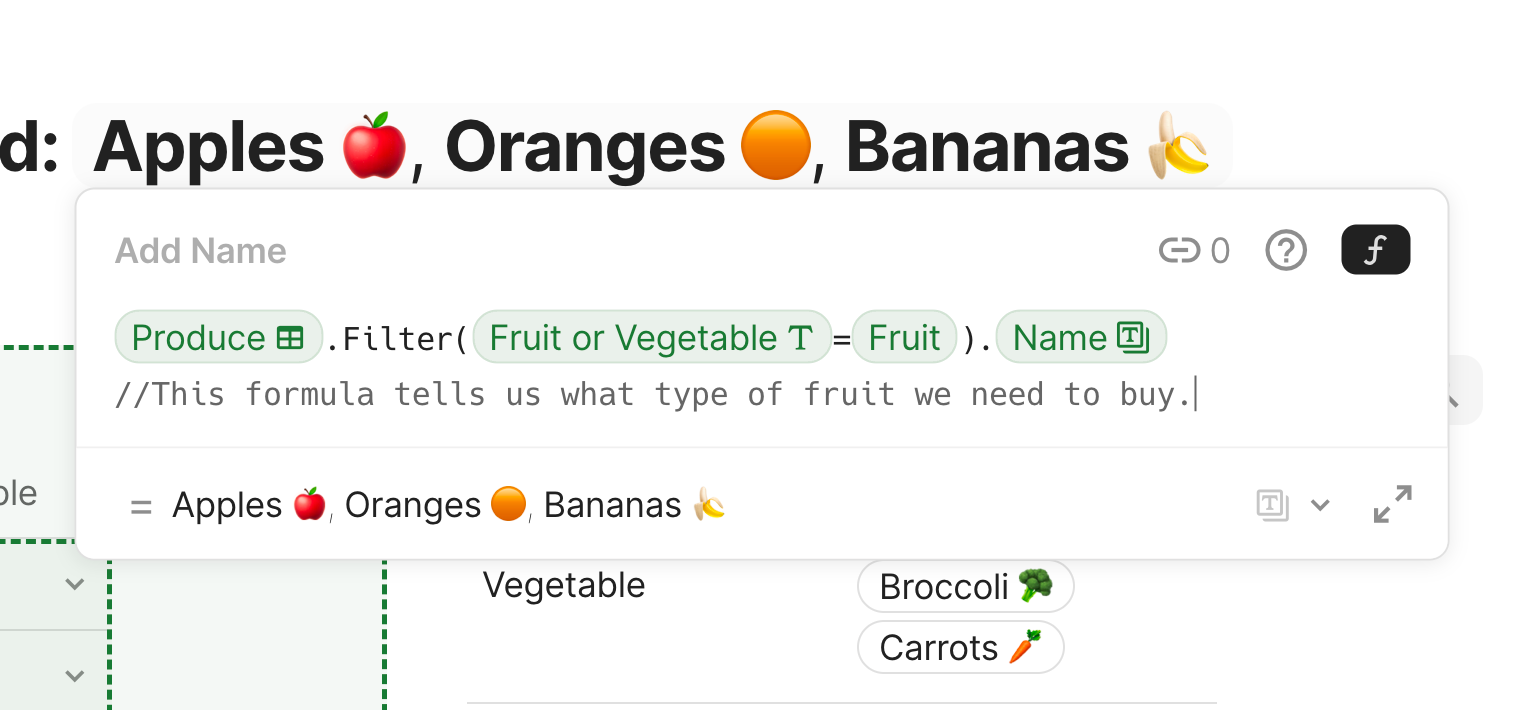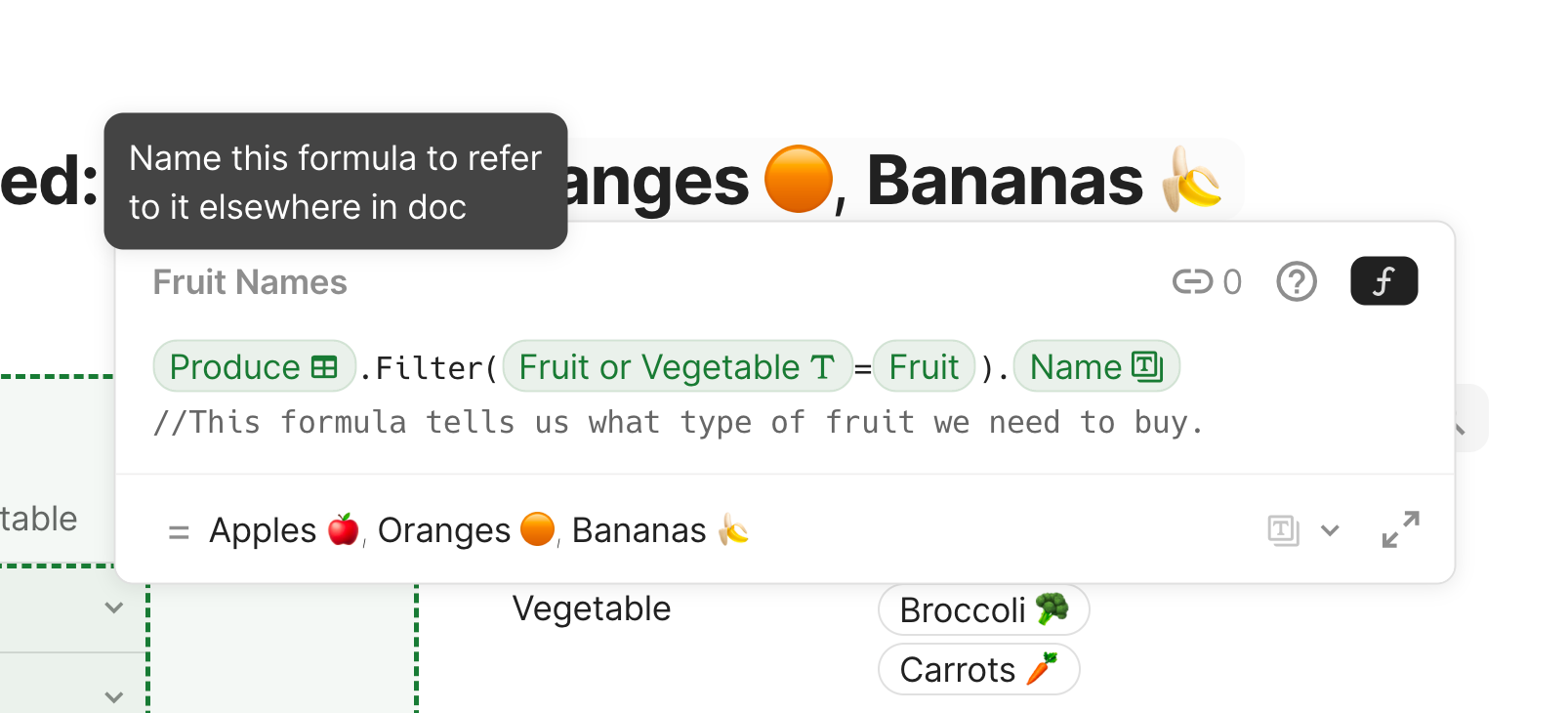# Writing a formula in Coda

Meet Coda's formula builder.

With hundreds of formulas to offer, the Coda formula language is the key to unlocking endless possibilities as you build out your docs. But how do you actually get the formulas into your docs and tables? This guide will introduce you to the Coda formula builder and get you up and running with writing your first formulas. If you’ve never written a formula before, be sure to check out this guide on Coda’s formula language first! 🚀

### What’s in hereWhat you'll get in this guide:
• An intro to Coda’s formula builder
• How to add formulas to the canvas
• How to add formulas to table columnsWhat you'll use in this guide:
• Formula builder
• Calculate
• Coda formula language

## 1. The formula builder.

Coda’s formula builder is where you will write all your formulas. You’ll access the formula builder in two main ways— directly on the canvas or in a table column.

### To open the formula builder on the canvas...

• Click anywhere on your page and type "`=`"
Tip: Trying to type "`=`" but not open the formula builder? Press "`Esc`" on your keyboard to exit the formula builder and keep the "`=`" character on your canvas.

### To open the formula builder in a specific table column...

• Find the column to which you want to add a formula.
• Hover over the column header and click on the column type icon to open the column settings. You can also right-click the column header to open the column settings.
• Click calculate to open up the formula builder for that specific column. You can choose to calculate from column data already present in your table or add a custom formula.
Tip: Once your cursor is inside the formula builder, you do not need to type "`=`" at the beginning of your formula.

## 2. Auto-suggestions, formula details, and errors.

Coda’s formula builder makes writing formulas as easy as possible for you. Here are a few cool things within the formula builder that will help you write great formulas for your docs:

### Auto-suggestions

As you write your formula in the formula builder, Coda will provide you with auto-suggestions for the data you might be trying to target with your formula.### Formula details

To the right of the list of auto-suggestions, if you select a formula rather than a table or column, you can access the details of a formula, like the correct inputs, expected outputs, and examples of how to use them.

### Formula errors

If you write a formula that won’t compute, the formula builder will let you know where the error is and what might be missing. Look for the red squiggle lines you might find under a misspelled word and the error code displayed in the formula builder. Check out this article for more tips on troubleshooting your formulas.

## 3. Expanding the editor and auto-formatting.

If you’re writing a formula and feel like you want a little more space to work, you can expand the formula builder to a modal view:
• Open the formula builder.
• Click the Expand icon in the bottom-right corner of the formula builder.
In the expanded view modal, you now have the option to auto-format your formula for clarity. To do so, click auto-format in the top-right corner of the expanded formula builder modal.

In the formula builder, use `//` before one-line comments, or wrap your longer (more than one line) comments in `/*` and `*/` `// This is an example of a one line comment.` `/* This is an example of a much longer comment. These are great to use to help others in your doc understand what a particular formula is doing, or to simply remind yourself while you learn! */`## 5. Name formulas you’ll use more than once.

As you start building out your docs, you may find that you want to use one of your handy formulas in multiple places. Rather than having to copy/paste or remember your work, you can name a formula and use it like a doc variable. You can name your formula by clicking Add Name in the formula builder right above where you’ve written your formula.## Now what?

Now that you’re familiar with the formula builder and ready to add in formulas for everything, be sure to check out these other resources to keep flexing those formula muscles! 💪 🧠